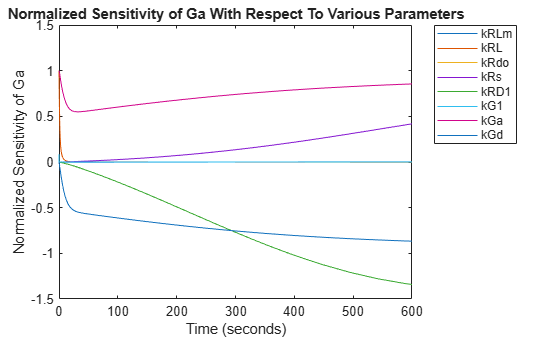# Calculate Sensitivities Using sbiosimulate

This example performs local sensitivity analysis on a Model of the Yeast Heterotrimeric G Protein Cycle to find parameters that influence the amount of active G protein. Assume that you are calculating the sensitivity of species Ga with respect to every parameter in the model. Thus, you want to calculate the time-dependent derivatives:

`$\frac{\partial \left(Ga\right)}{\partial \left(kRLm\right)},\frac{\partial \left(Ga\right)}{\partial \left(kRL\right)},\frac{\partial \left(Ga\right)}{\partial \left(kG1\right)},\frac{\partial \left(Ga\right)}{\partial \left(kGa\right)}...$`

The provided SimBiology project `gprotein_norules.sbproj` contains a model that represents the wild-type strain (stored in variable `m1`).

`sbioloadproject gprotein_norules.sbproj m1`

### Set up Sensitivity Analysis Options

The options for sensitivity analysis are in the `configset` object of the model.

`csObj = getconfigset(m1);`

Use the `sbioselect` function, which lets you query by type, to retrieve the `Ga` species from the model.

`Ga = sbioselect(m1,'Name','Ga');`

Set the `Outputs` property of the `SensitivityAnalysisOptions` object to the `Ga` species.

`csObj.SensitivityAnalysisOptions.Outputs = Ga;`

Retrieve all the parameters from the model and store the vector in a variable, `pif`.

`pif = sbioselect(m1,'Type','parameter');`

Set the `Inputs` property of the `SensitivityAnalysisOptions` object to the `pif` variable containing the parameters.

`csObj.SensitivityAnalysisOptions.Inputs = pif;`

Enable sensitivity analysis in the `configset` object by setting the `SensitivityAnalysis` option to `true`.

`csObj.SolverOptions.SensitivityAnalysis = true;`

Set the `Normalization` property of the `SensitivityAnalysisOptions` object to perform `'Full'` normalization.

`csObj.SensitivityAnalysisOptions.Normalization = 'Full';`

### Calculate Sensitivities

Simulate the model and return the data to a `SimData object`:

`simDataObj = sbiosimulate(m1);`

### Extract and Plot Sensitivity Data

You can extract sensitivity results using the `getsensmatrix` method of a `SimData object`. In this example, `R` is the sensitivity of the species `Ga` with respect to eight parameters. This example shows how to compare the variation of sensitivity of `Ga` with respect to various parameters, and find the parameters that affect `Ga` the most.

`[T, R, snames, ifacs] = getsensmatrix(simDataObj);`

Because `R` is a 3-D array with dimensions corresponding to times, output factors, and input factors, reshape `R` into columns of input factors to facilitate visualization and plotting:

`R2 = squeeze(R);`

After extracting the data and reshaping the matrix, plot the data:

```figure; plot(T,R2); title('Normalized Sensitivity of Ga With Respect To Various Parameters'); xlabel('Time (seconds)'); ylabel('Normalized Sensitivity of Ga'); leg = legend(ifacs, 'Location', 'NorthEastOutside'); set(leg, 'Interpreter', 'none');```From the plot you can see that `Ga` is most sensitive to parameters `kGd`, `kRs`, `kRD1`, and `kGa`. This suggests that the amounts of active G protein in the cell depends on the rate of:

• Receptor synthesis

• Degradation of the receptor-ligand complex

• G protein activation

• G protein inactivation